# User:IssaRice/Linear algebra/Matrix of a linear transformation

Axler writes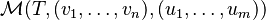$\mathcal M(T, (v_1,\ldots, v_n), (u_1, \ldots, u_m))$. Axler never abbreviates bases, although if he did, the notation would look like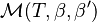$\mathcal M(T, \beta, \beta')$ where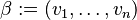$\beta := (v_1,\ldots, v_n)$ and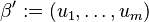$\beta' := (u_1, \ldots, u_m)$.
Tao writes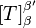$[T]_\beta^{\beta'}$, where the "in" basis is a subscript and the "out" basis is a superscript. With vectors,$[v]_\beta$ is a row vector and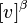$[v]^\beta$ is a column vector. This means that we can write$[T]_\beta^{\beta'} [v]^\beta = [v]^{\beta'}$ and think of the lower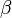$\beta$ canceling out the upper$\beta$.
Treil writes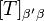$[T]_{\beta'\beta}$ or sometimes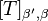$[T]_{\beta',\beta}$. With vectors, we can write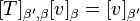$[T]_{\beta',\beta} [v]_\beta = [v]_{\beta'}$ so that the bases "balance".